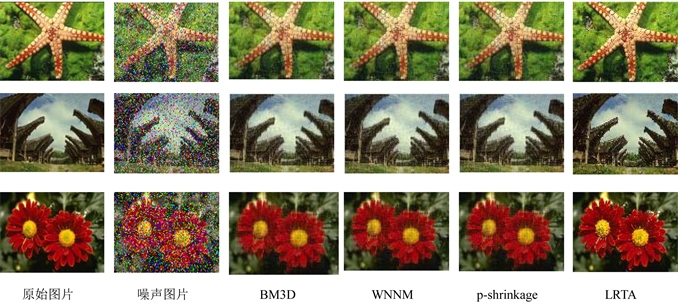﻿ 改进的低秩张量逼近算法

# 改进的低秩张量逼近算法Enhanced Low Rank Tensor Approximation Algorithm

Abstract: In this paper, we extended the low rank matrix approximation problem to tensors. A method for estimating low-rank tensors by constructing convex optimization problems with non-convex reg-ularization is proposed. The non-zero singular values are estimated by parameterized non-convex penalty functions, and the global optimal solution of the objective function is obtained. The ex-perimental results show that this method can deal with the low rank tensor approximation problem very well and achieve image denoising.

1. 引言

2. 预备知识

$bcirc\left(\mathcal{A}\right)=\left[\begin{array}{cccc}{A}^{\left(1\right)}& {A}^{\left({n}_{3}\right)}& \cdots & {A}^{\left(2\right)}\\ {A}^{\left(2\right)}& {A}^{\left(1\right)}& \cdots & {A}^{\left(3\right)}\\ ⋮& ⋮& \ddots & ⋮\\ {A}^{\left({n}_{3}\right)}& {A}^{\left({n}_{3}-1\right)}& \cdots & {A}^{\left(1\right)}\end{array}\right]\in {C}^{{n}_{1}{n}_{3}×{n}_{2}{n}_{3}}$ (1)

$\stackrel{¯}{A}=bdiag\left(\stackrel{¯}{\mathcal{A}}\right)=\left[\begin{array}{cccc}{\stackrel{¯}{A}}^{\left(1\right)}& & & \\ & {\stackrel{¯}{A}}^{\left(2\right)}& & \\ & & \ddots & \\ & & & {\stackrel{¯}{A}}^{\left({n}_{3}\right)}\end{array}\right]\in {C}^{{n}_{1}{n}_{3}×{n}_{2}{n}_{3}}$ (2)

${‖\mathcal{A}‖}_{\ast }=〈\mathcal{S},\mathcal{I}〉=\underset{i=1}{\overset{r}{\sum }}\mathcal{S}\left(i,i,1\right)$ (3)

1) $\varphi$ 在R上连续并且 $\varphi$$R/\left\{0\right\}$ 上是连续可微的且为偶函数即 $\varphi \left(-x;a\right)=\varphi \left(x;a\right)$

2) ${\varphi }^{\prime }\left(x;a\right)\ge 0,\forall a>0$

3) ${\varphi }^{″}\left(x;a\right)\le 0,\forall a>0$ (4)

4) ${\varphi }^{\prime }\left({0}^{-};a\right)=1$

5) $\underset{X\ne 0}{\mathrm{inf}}{\varphi }^{″}\left(x;a\right)={\varphi }^{″}\left({0}^{+};a\right)=-a$

$\mathcal{A}=\mathcal{U}\ast \mathcal{S}\ast {\mathcal{V}}^{\ast }$ (5)

$\stackrel{¯}{X}=U\cdot \Theta \left(\Sigma ;\lambda ,a\right)\cdot {V}^{\text{T}}$ (6)

3. 改进的低秩张量逼近算法

3.1. 模型的建立

$\mathcal{Y}$ 为受到噪声污染后的低秩张量， $\mathcal{X}$ 为潜在的目标低秩张量， $\mathcal{E}$ 为高斯噪声张量。则有 $\mathcal{Y}=\mathcal{X}+\mathcal{E}$ 。所以低秩张量逼近模型如下：

$\underset{\mathcal{X}}{\mathrm{arg}\mathrm{min}}\left\{\Psi \left(\mathcal{X}\right)=\frac{1}{2}{‖\mathcal{Y}-\mathcal{X}‖}_{F}^{2}+\lambda \underset{i=1}{\overset{k}{\sum }}\varphi \left(\mathcal{S}\left(i,i,1\right),a\right)\right\}$ (7)

3.2. 模型的求解

$\frac{1}{2}\left[\frac{1}{{n}_{3}}{‖\stackrel{¯}{Y}‖}_{F}^{2}+{‖\stackrel{¯}{X}‖}_{F}^{2}-2\cdot \frac{1}{{n}_{3}}〈\stackrel{¯}{Y},\stackrel{¯}{X}〉\right]+\lambda \varphi \left({‖\mathcal{X}‖}_{\ast },a\right)$ (9)

$\begin{array}{l}\frac{1}{2{n}_{3}}{‖\stackrel{¯}{Y}-\stackrel{¯}{X}‖}_{F}^{2}+\frac{1}{{n}_{3}}\lambda \varphi \left({‖\stackrel{¯}{X}‖}_{\ast },a\right)\\ =\frac{1}{{n}_{3}}\left[\frac{1}{2}{‖\stackrel{¯}{Y}-\stackrel{¯}{X}‖}_{F}^{2}+\lambda \underset{i=1}{\overset{k}{\sum }}\varphi \left({\sigma }_{i}\left(\stackrel{¯}{X}\right),a\right)\right]\end{array}$ (10)

$\frac{1}{{n}_{3}}\left(U\cdot \Theta \left(\Sigma ;\lambda ,a\right)\cdot {V}^{\text{T}}\right)$ (11)

4. 实验

${\stackrel{^}{\mathcal{X}}}_{j}:=\underset{\mathcal{X}}{\mathrm{arg}\mathrm{min}}\left\{\frac{1}{2}{‖{\mathcal{Y}}_{j}-\mathcal{X}‖}_{F}^{2}+\lambda \underset{i=1}{\overset{m}{\sum }}\varphi \left(S\left(i,i,1\right);a\right)\right\}$ (10)Figure 1. Comparison of denoising effects of different methodsTable 1. PSNR values and run time data

5. 结论

 Nadakuditi, R.R. (2014) OptShrink: An Algorithm for Improved Low-Rank Signal Matrix Denoising by Optimal, Da-ta-Driven Singular Value Shrinkage. IEEE Transactions on Information Theory, 60, 3002-3018.
https://doi.org/10.1109/TIT.2014.2311661

 Markovsky, I. (2008) Structured Low-Rank Approximation and Its Applications. Automatica, 44, 891-909.
https://doi.org/10.1016/j.automatica.2007.09.011

 Nguyen, H.M., Peng, X., Do, M.N. and Liang, Z.P. (2013) Denoising MR Spectroscopic Imaging Data with Low-Rank Approximations. IEEE Transactions on Biomedical Engi-neering, 60, 78-89.
https://doi.org/10.1109/TBME.2012.2223466

 Drineas, P., Kannan, R., and Mahoney, M.W. (2006) Fast Monte Carlo Algorithms for Matrices II: Computing a Low-Rank Approximation to a Matrix. SIAM Journal on com-puting, 36, 158-183.
https://doi.org/10.1137/S0097539704442696

 Kannan, R. and Vempala, S. (2009) Spectral Algorithms. Foundations and Trends in Theoretical Computer Science, 4, 157-288.
https://doi.org/10.1561/0400000025

 Candes, E.J. and Recht, B. (2008) Exact Low-Rank Matrix Completion via Convex Optimization. 2008 46th Annual Allerton Conference on Communication, Control, and Computing, Urba-na-Champaign, IL, 23-26 September 2008, 806-812.
https://doi.org/10.1109/ALLERTON.2008.4797640

 Cai, J.F., Candes, E.J. and Shen, Z.W. (2010) A Singular Value Thresholding Algorithm for Matrix Completion. SIAM Journal on Optimization, 20, 1956-1982.
https://doi.org/10.1137/080738970

 Lu, C., Feng, J., Chen, Y., Liu, W., Lin, Z. and Yan, S. (2018) Tensor Robust Principal Component Analysis with a New Tensor Nuclear Norm. IEEE Transactions on Pattern Analysis and Machine Intelligence, 1.
https://doi.org/10.1109/TPAMI.2019.2891760

 Parekh, A. and Selesnick, I.W. (2016) Enhanced Low-Rank Matrix Approximation. IEEE Signal Processing Letters, 23, 493-497.
https://doi.org/10.1109/LSP.2016.2535227

Top